## How to Calculate and Solve for the Volume of a Spherical Segment | Nickzom Calculator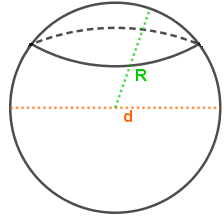The image above is a spherical segment.

To compute the volume of a spherical segment requires three essential parameters which are the radius of the spherical segment base (r1), radius of the spherical segment (r2) and height (h).

The formula for calculating the volume of the spherical segment:

V = πh(3r1² + 3r2² + h²)6

Where;
V = Volume of the spherical cap
r1 = Radius of the spherical segment base
r2 = Radius of the spherical segment base
h = Height of the spherical segment

Let’s solve an example;
Find the volume of a spherical segment when the radius of the spherical segment base (r1) is 7 cm, radius of the spherical segment base (r2) is 9 cm and a height of 20 cm.

This implies that;
r1 = Radius of the spherical segment base = 7 cm
r2 = Radius of the spherical segment base = 9 cm
h = Height of the spherical segment = 20 cm

V = πh(3r1² + 3r2² + h²)6
V = π x 20(3 x 7² + 3 x 9² + 20²)6
V = π x 20(3 x 49 + 3 x 81 + 400)6
V = π x 20(147 + 243 + 400)6
V = π x 20(790)6
V = π x 158006
V = 49643.66
V = 8273.9

Therefore, the volume of the spherical segment is 8273.9 cm3.

## How to Calculate and Solve for the Volume of a Spherical Cap | Nickzom Calculator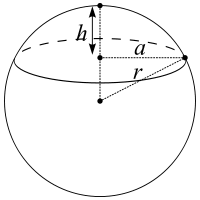The image above is a spherical cap.

To compute the volume of a spherical cap requires two essential parameters which are the radius of the base of the cap (a) and the height (h).

The formula for calculating the volume of the spherical cap:

V = πh(3a² + h²) ⁄ 6

Where;
V = Volume of the spherical cap
a = Radius of the base of the cap
h = Height of the spherical cap

Lets solve an example;
Find the volume of a spherical cap when the radius of the base is 12 cm and the height is 22 cm.

This implies that;
a = Radius of the base of the cap = 12 cm
h = Height of the spherical cap = 22 cm

V = πh(3a² + h²) ⁄ 6
V = 3.142 (22)(3(12)² + 22²) ⁄ 6
V = 3.142 (22)(3(144) + 484) ⁄ 6
V = 3.142 (22)(432 + 484) ⁄ 6
V = 3.142 (22)(916) ⁄ 6
V = 3.142 (20152)6
V = 63309.37 ⁄ 6
V = 10551.56

Therefore, the volume of the spherical cap is 10551.56 cm2.

## How to Calculate and Solve for the Width, Height, Length and Volume of a Rectangular Prism | Nickzom Calculator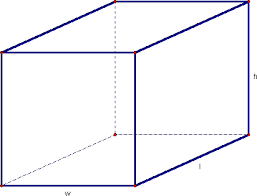The image above is a rectangular prism.

To compute the volume of a rectangular prism requires three essential parameters which are the length, width and height of the rectangular prism.

V = lwh

Where;
V = Volume of the rectangular prism
l = Length of the rectangular prism
w = Width of the rectangular prism
h = Height of the rectangular prism

Let’s solve an example;
Find the volume of a rectangular prism with a length of 18 cm, a width of 12 cm and a height of 21 cm.

This implies that;
l = Length of the rectangular prism = 18 cm
w = Width of the rectangular prism = 12 cm
h = Height of the rectangular prism = 21 cm

V = lwh
V = 18 x 12 x 21
V = 4536

Therefore, the volume of the rectangular prism is 4536 cm3.

Calculating the Length of a Rectangular Prism using the Volume, Width and Height of the Rectangular Prism.

l = V / wh

Where;
l = Length of the rectangular prism
V = Volume of the rectangular prism
w = Width of the rectangular prism
h = Height of the rectangular prism

Let’s solve an example;
Find the length of a rectangular prism with a width of 19 cm, a height of 24 cm and a Volume of 250 cm3.

This implies that;
w = Width of the rectangular prism = 19 cm
h = Height of the rectangular prism = 24 cm
V = Volume of the rectangular prism = 250 cm3

l = V / wh
l = 250 / 19 x 24
l = 250 / 456
l = 0.55

Therefore, the length of the rectangular prism is 0.55 cm.

## How to Calculate and Solve for the Height, Base Edge and Volume of a Square Pyramid | The Calculator Encyclopedia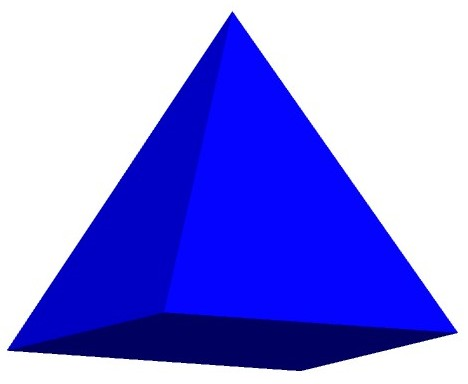The image above represents a square pyramid.
To compute the volume of a square pyramid requires two essential parameters which are the base edge and height of the square pyramid.

The formula for computing the volume of a square pyramid is:

V = ha² / 3

Where:
V = Volume of the Square Pyramid
a = Base edge of the Square Pyramid
h = Height of the Square Pyramid

Let’s solve an example
Find the volume of a square pyramid with a base edge of 6 cm and a height of 11 cm.

This implies that:
a = base edge of the square pyramid = 6
h= = height of the square pyramid = 11

V = ha² / 3
V = 11(6)² / 3
V = 11(36) / 3
V = 396 / 3
V = 132

Therefore, the volume of the square pyramid is 132 cm3.

Calculating the Base edge of a square pyramid when Volume and Height are Given

The formula is a = √(3V / h)

Where;
V = Volume of the Square Pyramid
a = Base edge of the Square Pyramid
h = Height of the Square Pyramid

Let’s solve an example:
Find the base edge of a square pyramid with a volume of 50 cm3 and a height of 20 cm.

This implies that;
V = Volume of the square pyramid = 50 cm3
h  = height of the square pyramid = 20 cm

a = √(3V / h)
a = √(3(50) / 20)
a = √(150 / 20)
a = √7.5
a = 2.739

Therefore, the base edge of the square pyramid is 2.739 cm.

Calculating the height of a square pyramid when Volume and Base edge are Given

The formula is h = 3V / a2

Where;
a = Base edge of the square pyramid
V = Volume of the Square Pyramid
h = Height of the Square Pyramid

Let’s solve an example:
Find the height of a square pyramid with a volume of  250 cm3  and a base edge of 7 cm2

This implies that;
V = Volume of the square pyramid = 250 cm3
a = Base edge of the square pyramid = 7 cm

h =  3V /
h = 3(250) /
h = 750 / 49
h = 15.306

Therefore, the height of the square pyramid is 15.306 cm.

## How to Calculate the Volume, Base Area and Height of a Prism | The Calculator Encyclopedia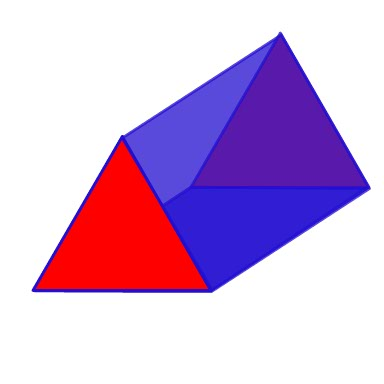The image above represents a prism.
To compute the volume of a prism requires two essential parameters which are the base area and height of the prism.

The formula for computing the volume of a prism is:

V = Ah

Where:
V = Volume of the Prism
A = Base area of the Prism
h = Height of the prism

Let’s solve an example
Find the volume of a prism with a base area of 30 cm2 and a height of 8 cm.

This implies that:
A = base area of the prism = 30
h= = height of the prism = 8

V = Ah
V = 30 x 8
V = 240

Therefore, the volume of the prism is 240 cm3.

Calculating the Base area of a prism when Volume and Height are Given

The formula is A = V / h

Where;
V = Volume of the Prism
A = Base area of the Prism
h = Height of the Prism

Let’s solve an example:
Find the base area of a prism with a volume of 300 cm3 and a height of 12 cm.

This implies that;
V = Volume of the prism = 300 cm3
h  = height of the prism = 12 cm

A =  V / h
A = 300 / 12
A = 25

Therefore, the base area of the prism is 25 cm2.

Calculating the height of a prism when Volume and Base area are Given

The formula is h = V / A

Where;
A = Base area of the Prism
V = Volume of the Prism
h = Height of the Prism

Let’s solve an example:
Find the height of a prism with a volume of  280 cm3  and a base area of 35 cm2

This implies that;
V = Volume of the prism = 280 cm3
A = Base area of the prism = 35 cm2

h =  V / A
h = 280 / 35
h = 8

Therefore, the height of the prism is 8 cm.

## How to Calculate the Volume, Length, Width and Height of a Cuboid | Nickzom Calculator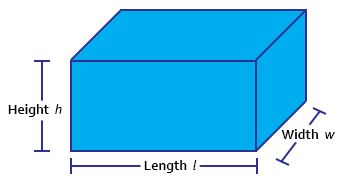The image above represents a cuboid.
To compute the volume of a cuboid requires three essential parameters which are the length, width and height of the cuboid.

The formula for computing the volume of a cuboid is:

V = lwh

Where:
V = Volume of the Cuboid
l = Length of the Cuboid
w = Width of the Cuboid
h = Height of the Cuboid

Let’s solve an example
Find the volume of a cuboid with a length of 6 cm, width of 2 cm and a height of 10 cm.

This implies that:
l = length of the cuboid = 6
w = width of the cuboid = 2
h= = height of the cuboid = 10

V = lwh
V = 6 x 2 x 10
V = 120

Therefore, the volume of the cuboid is 120 cm3.

Calculating the Length of a cuboid when Volume, Width and Height are Given

The formula is l = V / (w)(h)

Where;
V = Volume of the Cuboid
l = Length of the Cuboid
w = Width of the Cuboid
h = Height of the Cuboid

Let’s solve an example:
Find the length of a cuboid with a volume of 440 cm3 , a width of 5 cm and a height of 11 cm.

This implies that;
V = Volume of the cuboid = 440 cm3
w = width of the cuboid = 5 cm
h  = height of the cuboid = 11 cm

l =  V / (w)(h)
l = 440 / (5)(11)
l = 440 / 55
l = 8 cm

Therefore, the length of the cuboid is 8 cm.

Calculating the Width of a cuboid when Volume, Length and Height are Given

The formula is w = V / (l)(h)

Where;
V = Volume of the Cuboid
l = Length of the Cuboid
w = Width of the Cuboid
h = Height of the Cuboid

Let’s solve an example:
Find the width of a cuboid with a volume of  180 cm3 , a length of 6 cm and a height of 10 cm

This implies that;
V = Volume of the cuboid = 180 cm3
l = length of the cuboid = 6 cm
h  = height of the cuboid = 10 cm

w =  V / (l)(h)
w = 180 / (6)(10)
w = 180 / 60
w = 3 cm

Therefore, the width of the cuboid is 3 cm.

Calculating the Height of a cuboid when Volume, Length and Width are Given

The formula is h = V / (l)(w)

Where;
V = Volume of the Cuboid
l = Length of the Cuboid
w = Width of the Cuboid
h = Height of the Cuboid

Let’s solve an example:
Find the height of a cuboid with a volume of 195 cm3 , a length of 5 cm and a width of 3 cm

This implies that;
V = Volume of the cuboid = 195 cm3
l = length of the cuboid = 5 cm
w  = width of the cuboid = 3 cm

h =  V / (l)(w)
h = 195 / (5)(3)
h = 195 / 15
h = 13 cm

Therefore, the height of the cuboid is 13 cm.

## How to Calculate and Solve for the Volume and Radius of a Sphere | The Calculator Encyclopedia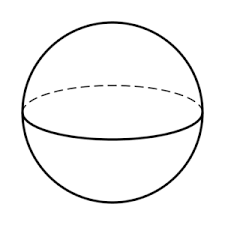The image above is a sphere.

To compute the volume of a sphere, one essential parameter is needed and this parameter is the radius of the sphere (r). You can also use diameter of the sphere (d).

The formula for calculating the volume of a sphere:

V = (4/3)πr³

Where;

V = Volume of the sphere
r = Radius of the sphere

Let’s solve an example:
Find the volume of a sphere when the radius of the sphere is 10 cm.

This implies that;

r = Radius of the sphere = 10 cm

V = (4/3)πr³
V = (4/3)π x (10)³
V = (4/3)π x 1000
V = (4/3)3.142 x 1000
V = (4/3)3142
V = 1.33 x 3142
V = 4188.7

Therefore, the volume of the sphere is 4188.7 cm³.

Calculating the Volume of a Sphere using Diameter of the Sphere.

V = 4πd³ / 24

Where;

V = Volume of the sphere
d = Diameter of the sphere

Let’s solve an example:
Find the volume of a sphere when the diameter of the sphere is 20 cm.

This implies that;

d = Diameter of the sphere = 20 cm

V = 4πd³ / 24
V = 4 x 3.142 x (20)³ / 24
V = 4 x 3.142 x 8000 / 24
V = 100544 / 24
V = 4189.3

Therefore, the volume of the sphere with diameter is 4189.3 cm3.

Calculating the Radius of a Sphere using Volume of the Sphere.

r = 3√(3V / )

Where;

V = Volume of the sphere
r = Radius of the sphere

Let’s solve an example:
Find the radius of a sphere when the volume of the sphere is 250 cm3.

This implies that;

V = Volume of the sphere = 250 cm3

r = 3√(3V / )
r = 3√(3 x 250 / 4 x 3.142)
r = 3√(750 / 12.568)
r = 3√59.675
r = 3.907

Therefore, the radius of the sphere is 3.907 cm.

Calculating the Diameter of a Sphere using Volume of the Sphere.

d = 3√(24V / )

Where;

A = Area of the sphere
d = Diameter of the sphere

Let’s solve an example:
Find the diameter of a sphere when the volume of the sphere is 40 cm3.

This implies that;

V = Volume of the sphere = 40 cm3

d = 3√(24V / )
d = 3√(24 x 40 / 4 x 3.142)
d = 3√(960 / 12.568)
d = 3√76.38
d = 4.24

Therefore, the diameter of the sphere is 4.24 cm.

## How to Calculate and Solve for the Volume, Radius and Height of a Cylinder | Nickzom Calculator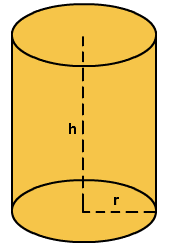The image above represents a cylinder.
To compute the volume of a cylinder requires two essential parameters which are the radius and height of the cylinder.

The formula for computing the volume of a cylinder is:

V = πr2h

Where:
V = Volume of a cylinder
r = radius of the cylinder
h = height of the cylinder

Let’s solve an example
Find the volume of a cylinder with a radius of 3 cm and a height of 5 cm.

This implies that:
r = radius of the cylinder = 3
h = height of the cylinder = 5

V = πr2h
V = 3.142 x 32 x 5
V = 141.39

Therefore, the volume of the cylinder is 141.39 cm3.

Calculating the Height of a cylinder when Volume and Radius is Given

The formula is h = V / πr2

Where;
V = Volume of a cylinder
r =  radius of the cylinder
h = height of the cylinder

Let’s solve an example:
Find the height of a cylinder with a volume of 300 cm3 and a radius of 3 cm

This implies that;
V = Volume of the cylinder = 300 cm3
r  = radius of the cylinder = 3 cm

h = V / πr2
h = 300 / 3.142(3)2
h = 300 / 28.278
h = 10.61
Therefore, the height of the cylinder is 10.61 cm.

Calculating the Radius of a cylinder when Volume and Height is Given

The formula is r = √(V / πh)

Where;
V = Volume of a cylinder
r =  radius of the cylinder
h = height of the cylinder

Let’s solve an example:
Find the radius of a cylinder with a volume of 200 cm3 and a height of 5 cm

This implies that;
V = Volume of the cylinder = 200 cm3
h  = height of the cylinder = 5 cm

r = √(V / πh)
r = √(200 / 3.142(5))
r = √(200 / 15.71)
r = √12.73
r = 3.57

Therefore, the radius of the cylinder is 3.57 cm.

## How to Calculate and Solve for the Volume and Length of a Cube | The Calculator Encyclopedia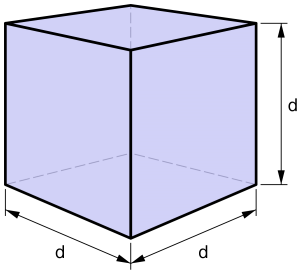The image above is a cube.

To compute the volume of a cube, one essential parameter is needed and this parameter is the length of the cube (l).

The formula for calculating the volume of a cube is;

V = l3

Where;

V = Volume of a cube
l = Length of a cube

Let’s solve an example:
Find the volume of a cube where the length of a cube is 4 cm.

This implies that;
l = length of the cube = 4 cm.

V = l3
V = 43
V = 64 cm3

Therefore, the volume of the cube is 64 cm3

Calculating the Length of a cube using the Volume of the cube.

The formula is l = 3√V

Where;

V = Volume of a cube
l = length of a cube

Let’s solve an example:
Find the length of a cube where the volume of the cube is 120 cm3

This implies that;
V = Volume of the cube = 120 cm3

l = 3√V
l = 3√120
l = 4.93

Therefore, the length of the cube is 4.93 cm.

## How to Calculate and Solve for Linear Expansivity, Area Expansivity and Volume or Cubic Expansivity

Linear expansivity, area expansivity and volume or cubic expansivity are all parameters that are being governed by heat energy. All three parameter is dependent on temperature rise which is primarily in Celsius unit of temperature.

Linear expansivity additionally depends on the original length and final length, area expansivity additionally depends on the original area and final area and lastly, cubic or volume expansivity depends on the original volume and final volume.

The formula for calculating linear expansivity is (l2 – l1) / l1θ
l2 represents the final length.
l1 represents the original length.
θ represents the temperature rise in Celsius.

The formula for calculating area expansivity is (A2 – A1) / A1θ
A2 represents the final area.
A1 represents the original area.
θ represents the temperature rise in Celsius.

The formula for calculating volume or cubic expansivity is (V2 – V1) / V1θ
V2 represents the final volume.
V1 represents the original volume.
θ represents the temperature rise in Celsius.